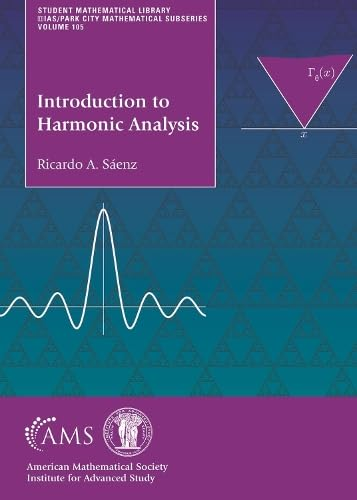# Introduction to Harmonic Analysis

• Length: 279 pages
• Edition: 1
• Publisher:
• Publication Date: 2023-06-29
• ISBN-10: 147047199X
• ISBN-13: 9781470471996
Description

This book gives a self-contained introduction to the modern ideas and problems of harmonic analysis. Intended for third- and fourth-year undergraduates, the book only requires basic knowledge of real analysis, and covers necessary background in measure theory, Lebesgue integration and approximation theorems. The book motivates the study of harmonic functions by describing the Dirichlet problem, and discussing examples such as solutions to the heat equation in equilibrium, the real and imaginary parts of holomorphic functions, and the minimizing functions of energy. It then leads students through an in-depth study of the boundary behavior of harmonic functions and finishes by developing the theory of harmonic functions defined on fractals domains. The book is designed as a textbook for an introductory course on classical harmonic analysis, or for a course on analysis on fractals. Each chapter contains exercises, and bibliographic and historical notes. The book can also be used as a supplemental text or for self-study.